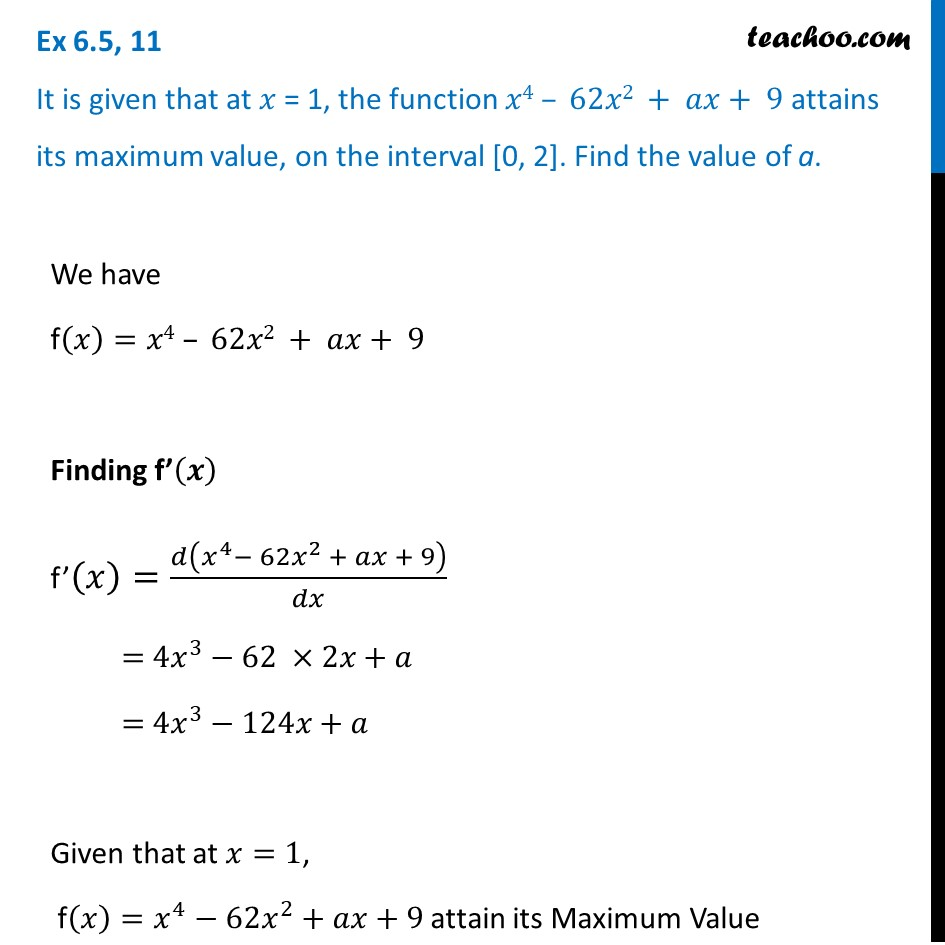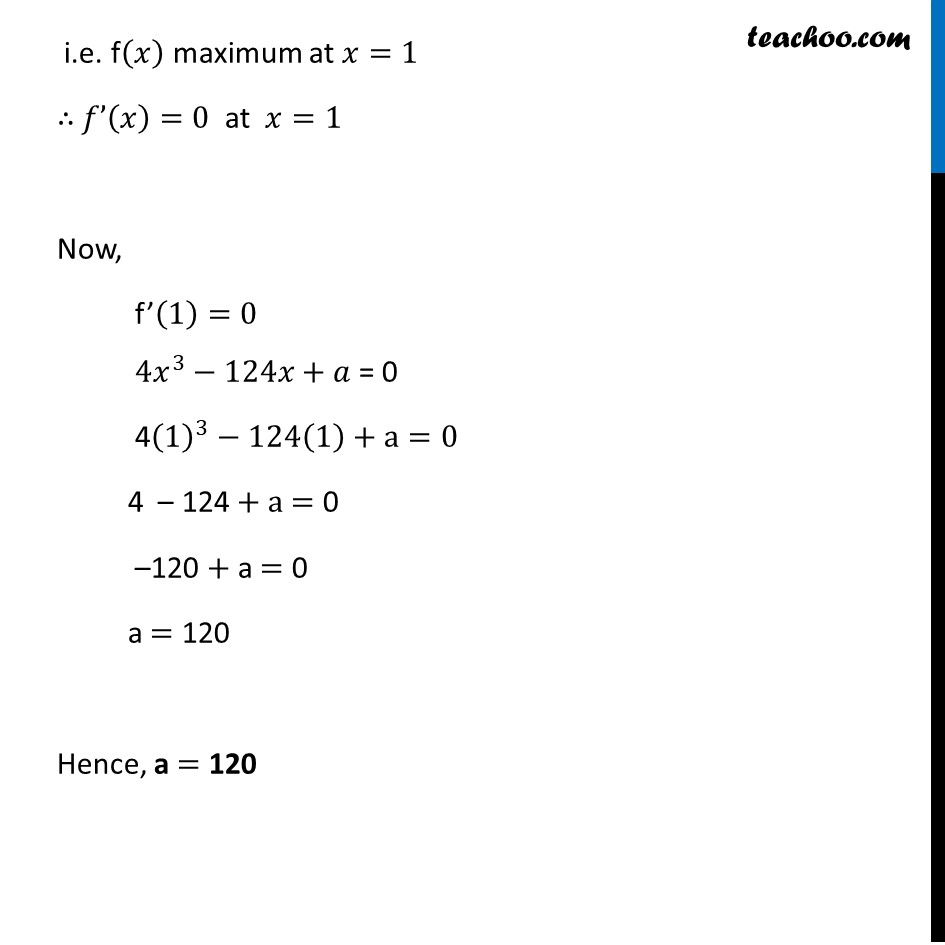Ex 6.5

Chapter 6 Class 12 Application of Derivatives
Serial order wiseGet live Maths 1-on-1 Classs - Class 6 to 12

### Transcript

Ex 6.5, 11 It is given that at 𝑥 = 1, the function 𝑥4 – 62𝑥2 + 𝑎𝑥+ 9 attains its maximum value, on the interval [0, 2]. Find the value of a.We have f(𝑥)=𝑥4 – 62𝑥2 + 𝑎𝑥+ 9 Finding f’(𝒙) f’(𝑥)=𝑑(𝑥^4− 62𝑥^2 + 𝑎𝑥 + 9)/𝑑𝑥 = 〖4𝑥〗^3−62 ×2𝑥+𝑎 = 〖4𝑥〗^3−124𝑥+𝑎 Given that at 𝑥=1, f(𝑥)=𝑥^4−62𝑥^2+𝑎𝑥+9 attain its Maximum Value i.e. f(𝑥) maximum at 𝑥=1 ∴ 𝑓’(𝑥)=0 at 𝑥=1 Now, f’(1)=0 〖4𝑥〗^3−124𝑥+𝑎 = 0 4(1)^3−124(1)+a=0 4 – 124 + a = 0 –120 + a = 0 a = 120 Hence, a = 120